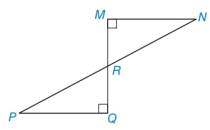Chapter 3.1, Problem 30E### Elementary Geometry for College St...

6th Edition
Daniel C. Alexander + 1 other
ISBN: 9781285195698

#### Solutions

Chapter
Section### Elementary Geometry for College St...

6th Edition
Daniel C. Alexander + 1 other
ISBN: 9781285195698
Textbook Problem
14 views

# In Exercises 27 to 32, use SSS, SAS, ASA, or AAS to prove that the triangles are congruent. Given: P N ¯ bisects M Q ¯ ∠ M and ∠ Q are right angles Prove: Δ P Q R ≅ Δ N M RTo determine

To prove:

The triangle ΔPQR is congruent to the triangle ΔNMR.

Explanation

Given:

The triangles ΔPQR and ΔNMR are shown in the figure below,

Figure (1)

From the figure (1),

PN¯ bisects MQ¯

And,

M and Q are right angles

Property used:

If two lines intersect, then the vertical angles formed are congruent.

Approach:

Consider the triangles,

ΔPQR and ΔNMR

From the given data,

The angles M and Q are right angles in triangles ΔPQR and ΔNMR.

Since, the angle M is equal to the angle Q, then

MQ

And the line PN¯ bisect the line MQ¯ in two equal lines MR¯ and RQ¯.

Since the lines MR¯ and RQ¯ are equal, then

MR¯RQ¯

Since, the line PN¯ bisect the line MQ¯, then there is vertical angles PRQ and MRN are formed

### Still sussing out bartleby?

Check out a sample textbook solution.

See a sample solution

#### The Solution to Your Study Problems

Bartleby provides explanations to thousands of textbook problems written by our experts, many with advanced degrees!

Get Started

#### Find the derivatives of the functions in Problems 1-34.

Mathematical Applications for the Management, Life, and Social Sciences

#### What is the value of (X + 1) for the following scores: 0, 1, 4, 2? a. 8 b. 9 c. 11 d. 16

Essentials of Statistics for The Behavioral Sciences (MindTap Course List)

#### Simplify: a2+3a4a2+2a3(a1,3)

College Algebra (MindTap Course List)